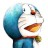# 杰拉斯的博客

## [ACM_HDU_1050]Moving Tables杰拉斯 | 时间：2012-04-30, Mon | 23,518 views

### Moving Tables

Time Limit: 2000/1000 MS (Java/Others) Memory Limit: 65536/32768 K (Java/Others)
Total Submission(s): 9950 Accepted Submission(s): 3389

#### Description

The famous ACM (Advanced Computer Maker) Company has rented a floor of a building whose shape is in the following figure.The floor has 200 rooms each on the north side and south side along the corridor. Recently the Company made a plan to reform its system. The reform includes moving a lot of tables between rooms. Because the corridor is narrow and all the tables are big, only one table can pass through the corridor. Some plan is needed to make the moving efficient. The manager figured out the following plan: Moving a table from a room to another room can be done within 10 minutes. When moving a table from room i to room j, the part of the corridor between the front of room i and the front of room j is used. So, during each 10 minutes, several moving between two rooms not sharing the same part of the corridor will be done simultaneously. To make it clear the manager illustrated the possible cases and impossible cases of simultaneous moving.For each room, at most one table will be either moved in or moved out. Now, the manager seeks out a method to minimize the time to move all the tables. Your job is to write a program to solve the manager’s problem.

#### Input

The input consists of T test cases. The number of test cases ) (T is given in the first line of the input. Each test case begins with a line containing an integer N , 1<=N<=200 , that represents the number of tables to move. Each of the following N lines contains two positive integers s and t, representing that a table is to move from room number s to room number t (each room number appears at most once in the N lines). From the N+3-rd line, the remaining test cases are listed in the same manner as above.

#### Output

The output should contain the minimum time in minutes to complete the moving, one per line.

```
3
4
10 20
30 40
50 60
70 80
2
1 3
2 200
3
10 100
20 80
30 50
```

```10
20
30
```

#### Source

HDU1050

```#include<stdio.h>
#include<vector>
#include<iterator>
#include<algorithm>
using namespace std;
struct M{
int s;
int t;
};

bool comp(const M &m1, const M &m2){
return m1.s < m2.s;	//按照开始的房间号排序
}

int main(){
int T, s, t;
scanf("%d", &T);
while(T--){
int n;
vector<M> v;
scanf("%d", &n);
for(int i = 0; i < n; ++i){
M m;
scanf("%d%d", &s, &t);
if(s > t){	//有可能从号码大的房间往回搬，若是，则交换s与t
s = s ^ t;	//交换s，t
t = s ^ t;	//相当于
s = s ^ t;	//int tmp = s; s = t; t = tmp;
}
m.s = s;
m.t = t;
v.push_back(m);
}
sort(v.begin(), v.end(), comp);
int ans = 0;
for(vector<M>::iterator it = v.begin(); it != v.end();){	//从房间号最小的开始查找
++ans;
int t = (*it).t;
for(vector<M>::iterator subit = it; subit != v.end();){	//找到与要搬的线路不冲突的桌子
if((*subit).s > t && !((*subit).s - t == 1 && (*subit).s % 2 == 0)){	//注意对面房间的线路也是冲突的，比如5与6
t = (*subit).t;
subit = v.erase(subit);	//该桌子已搬完，删除
}else
++subit;	//否则继续查找下一张桌子
}
it = v.erase(it);	//删除
}
printf("%d\n", ans * 10);
}
return 0;
}
```

```#include <iostream>
using namespace std;
int main()
{
int t, i, j, N, P;
int s, d, k, min;
cin >> t;
for(i = 0; i < t; ++i)
{
memset(P, 0, sizeof(P));
cin >> N;
for(j = 0; j < N; ++j)
{
cin >> s >> d;
s = (s - 1) / 2;
d = (d - 1) / 2;
if(s > d)
{
s = s ^ d;
d = s ^ d;
s = s ^ d;
}
for(k = s; k <= d; ++k)
++P[k];
}
min = -1;
for(j = 0; j < 200; ++j)
if(P[j] > min)
min = P[j];
cout << min * 10 << endl;
}
return 0;
}
```